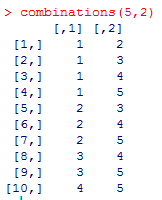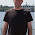## Monday, July 18, 2011

### Avoiding Loops in R: An Example with Principal Minors

Yesterday, I found myself wanting to compute a large subset of the second order principal minors of a matrix (diagonal-preserving minors; the ones for which the rows and columns kept are the same). Don't judge me for wanting to do this, and bear with me, there is a lesson about R programming here (mostly for me, read the comments for testing).

If you don't know what a principal minor is or forgot what it was (because most of us don't spend much time with principal minors), here is a short refresher on how to get a second-order principal minor (other orders are possible and there's a lot of related theory, but let's try to focus on this one task for now).

Step 1: Deletion. Take any matrix. Delete all but two rows and all but two columns. For example, suppose the matrix is 5x5 and let's keep rows 1 and 3 and columns 2 and 4 (lots of other combinations are possible).In the back of your mind, think how easy this is in R.

Step 2: Calculation. Once we delete the irrelevant parts of the big matrix to get our 2x2 submatrix, obtain the principal minor by taking the determinant of the resulting 2x2 matrix. For our example, we compute 3x2 - 3x1 = 3.

Both steps in this calculation are easy to implement in R. For the first step, merely use R's fantastic syntax for extracting rows and columns of matrices. If the matrix is called mat, we efficiently perform the first step by:

little_mat = mat[c(1,3), c(2,4)]

That's easy enough. With little_mat in hand, the second step is even easier.

pm = det(little_mat)

The real trick to this programming problem is picking out all of principal minors I want to compute (diagonal-preserving ones that keep the same-numbered rows and columns; little_mat won't do because c(1,3) is not c(2,4)), and doing it efficiently. I also don't want to rewrite my code as my matrix changes size.

So, what do we do? A really bad An idea that will eventually compute the right answer is to write this using for() loops.

Barring a coding error, this code should eventually work, but it will be slow (or fast depending on how you write it). If you want to run through this code many times (like I did yesterday), efficiency is at a premium and you should immediately think of faster techniques -- like using the apply() function (or preallocating your storage vector, which actually saves time).

Before moving to apply(), we need a quick way to enumerate all of the ways to keep two out of N rows. If we were counting, that's N choose 2, also known as a combination. Fortunately, this step has already been implemented in the gregmisc library with a function called combinations(). Conveniently, combinations(N, 2) will return a matrix with all of the ways to select two numbers less than or equal to N as its rows. There are other options, but this is the default, which is perfect for our application.

For example, here is some output for N=5.With this way of computing the rows/ columns we want to keep, all we need to do is put our simple code for computing a second order principal minor into a function. Let's call it minor2(). Then, use apply() to apply this function to each row (MARGIN=1 is for rows) of the combinations matrix. Here's the complete set of code that will work if you have a matrix called mat for which you want the diagonal-second order principal minors.

Because we avoid doing loops, this method is much faster. For a big project, this added efficiency is worth it. More generally, this technique of using apply() to avoid repeatedly looping is a good lesson to apply to other programming problems.

Update: Thanks to Berend for going through the trouble of system.time() testing the code in this post (and spiceman for a good comment as well). Read on into the comments for more, but for a preview of what we learned: (1) The copying via c() is what slows the loop down, (2) apply is slightly slower than writing the loop yourself, (3) combn(), the combinations() alternative, is really slow, and (4) one should always stand behind system.time() methods before talking about the efficiency of a method.

I much appreciate the feedback. One of the reasons I have this blog is to learn more about R (and those comments were definitely informative).

1.1. first for loop has an error and should read

for(i in 1:(nrow(mat)-1)){

2. why use gregmisc? You can use the R provided function combn and then use t(combn(nrow(mat),2)) to get the same result.

Berend

2.Thanks for the correction and the tip on combn. With regard to why to use gregmisc, I suppose that it depends on your application. It turns out that gregmisc is faster even without transposing (I tried that too).

> system.time(combinations(400,2))
user system elapsed
0.29 0.00 0.29
> system.time(t(combn(400,2)))
user system elapsed
1.04 0.00 1.05

But, gregmisc's combinations() function breaks on combinations much larger than this (I picked 1000 choose 2... which broke gregmisc's combinations, but not combn).

3.I tested as follows: number of replications N <- 1000

> system.time(replicate(N, {
+ for(i in 1:(nrow(mat)-1)){
+ start <- i + 1
+ for(j in start:nrow(mat)){
+ thisminor <- det(mat[c(i,j),c(i,j)])
+ minors.1 <- c(minors.1, thisminor)
+ }
+ }
+ }))
user system elapsed
0.417 0.001 0.421

> system.time(replicate(N, {
+ index_vec <- t(combn(nrow(mat),2))
+ minors.2 <- apply(index_vec, MARGIN=1, FUN=function(ix){ minor2(mat,ix)})
+ }))
user system elapsed
0.824 0.002 0.829

Even if you move the combn to before the second system.time the for loop is still faster albeit with a much smaller margin.

Conclusion: For loop is faster!

Berend

4.Thanks again for another challenging comment. Maybe I should not have been so unequivocal in my language about avoiding for loops. For small problems, they're fine.

I have been working with bigger matrices (on the order of 100 or 200 rows. In this setting, apply() saves time. I tested the code you provided (with combinations, rather than combn) as follows:

> mat = matrix(rnorm(200*200), nrow = 200)
> N = 25
>
> system.time(replicate(N,
+ {
+ minors.1 = NULL
+ for(i in 1:(nrow(mat)-1)){
+ start <- i + 1
+ for(j in start:nrow(mat)){
+ thisminor <- det(mat[c(i,j),c(i,j)])
+ minors.1 <- c(minors.1, thisminor)
+ }
+ }
+ }))
user system elapsed
30.75 0.03 30.79
>
>
> minor2 = function(mat, idx){
+ prin = mat[idx,idx]
+ return(det(prin))
+ }
>
> system.time(replicate(N, {
+ index_vec <- combinations(nrow(mat), 2)
+ minors.2 <- apply(index_vec, MARGIN=1, FUN=function(ix){ minor2(mat,ix)})
+ }))
user system elapsed
21.64 0.00 21.67

Conclusion: apply() scales better than the nested for loop. But, you're right. For smaller matrices, the for loop will be the faster option.

5.I think some speed would be gained by preallocating a vector of size nrow, so that R does not have to copy data by c(...).

minors[i] = thisminor

By the way, apply has an internal for loop...

6.Good point on preallocating the vector (copying slows R down quite a bit).

One caveat to your suggestion: The size you propose isn't right. There are usually many more minors of order two than rows in the matrix.

7.Some more tests.
I have tested with the following 4 methods

Method 1.
----------------------------

minors.1 <- NULL
system.time(replicate(N, {
for(i in 1:(nrow(mat)-1)){
start <- i + 1
for(j in start:nrow(mat)){
thisminor <- det(mat[c(i,j),c(i,j)])
minors.1 <- c(minors.1, thisminor)
}
}
}))

Method 2.
----------------------------

minors.1 <- numeric(nrow(mat)*nrow(mat)/2+nrow(mat)) #max
system.time(replicate(N, {
k <- 0
for(i in 1:(nrow(mat)-1)){
start <- i + 1
for(j in start:nrow(mat)){
thisminor <- det(mat[c(i,j),c(i,j)])
k <- k+1
minors.1[k] <- thisminor
}
}
}))

Method 3.
----------------------------

system.time(replicate(N, {
index_vec <- t(combn(nrow(mat),2))
minors.2 <- apply(index_vec, MARGIN=1, FUN=function(ix){ minor2(mat,ix)})
}))

Method 4.
----------------------------

index_vec <- t(combn(nrow(mat),2))
system.time(replicate(N, {
minors.2 <- apply(index_vec, MARGIN=1, FUN=function(ix){ minor2(mat,ix)})
}))

Timing results
------------------

N=25 nrow(mat)=200

Elapsed time
1. 55.862
2. 21.816
3. 33.192
4. 25.049

N=100 nrow(mat)=100

Elapsed time
1. 26.007
2. 21.968
3. 33.569
4. 24.758

In both these cases the method 2 appears to be the quickest.
So I would conclude that the for loop with preallocation of result vector
is likely to be the quickest.

The R provided function combn is also a bit of a bottleneck as can seen from the timings for method 3 and 4.
Executing combn once helps but the for loop with preallocation remains the quickest in the cases presented here.

Berend

8.On my system, this one-liner is a bit quicker:

> system.time(replicate(N, {
+ minors <- combn(nrow(mat), 2, function(x) {det(mat[x, x])})
+ }))
user system elapsed
35.758 0.000 35.763

And here are the results of Berend's Method 2:

> system.time(replicate(N, {
+ index_vec <- t(combn(nrow(mat),2))
+ minors.2 <- apply(index_vec, MARGIN=1, FUN=function(ix){minor2(mat,ix)})
+ }))
user system elapsed
41.755 0.024 41.810
>